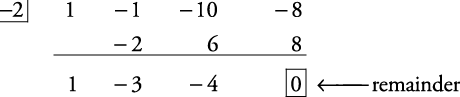## Factor Theorem

If P( x) is a polynomial, then P( r) = 0 if and only if xr is a factor of P( x).

##### Example 1

Is ( x + 2) a factor of x 3x 2 – 10 x – 8?

Check to see whether ( x 3x 2 – 10 x – 8) ÷ ( x + 2) has a remainder of zero. Using synthetic division, you getBecause the remainder of the division is zero, ( x + 2) is a factor of x 3x 2 – 10 x – 8. The expression x 3x 2 – 10 x – 8 can now be expressed in factored form.

x 3x 2 – 10 x – 8 = ( x + 2)( x 2 – 3 x – 4)

But ( x 2 – 3 x – 4) can be factored further into ( x – 4)( x + 1). So

x 3x 2 – 10 x – 8 = ( x + 2)( x – 4)( x + 1)

The expression x 3x 2 – 10 x – 8 is now completely factored. From this form, it also is seen that ( x – 4) and ( x + 1) are also factors of x 3x 2 – 10 x – 8.